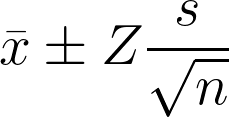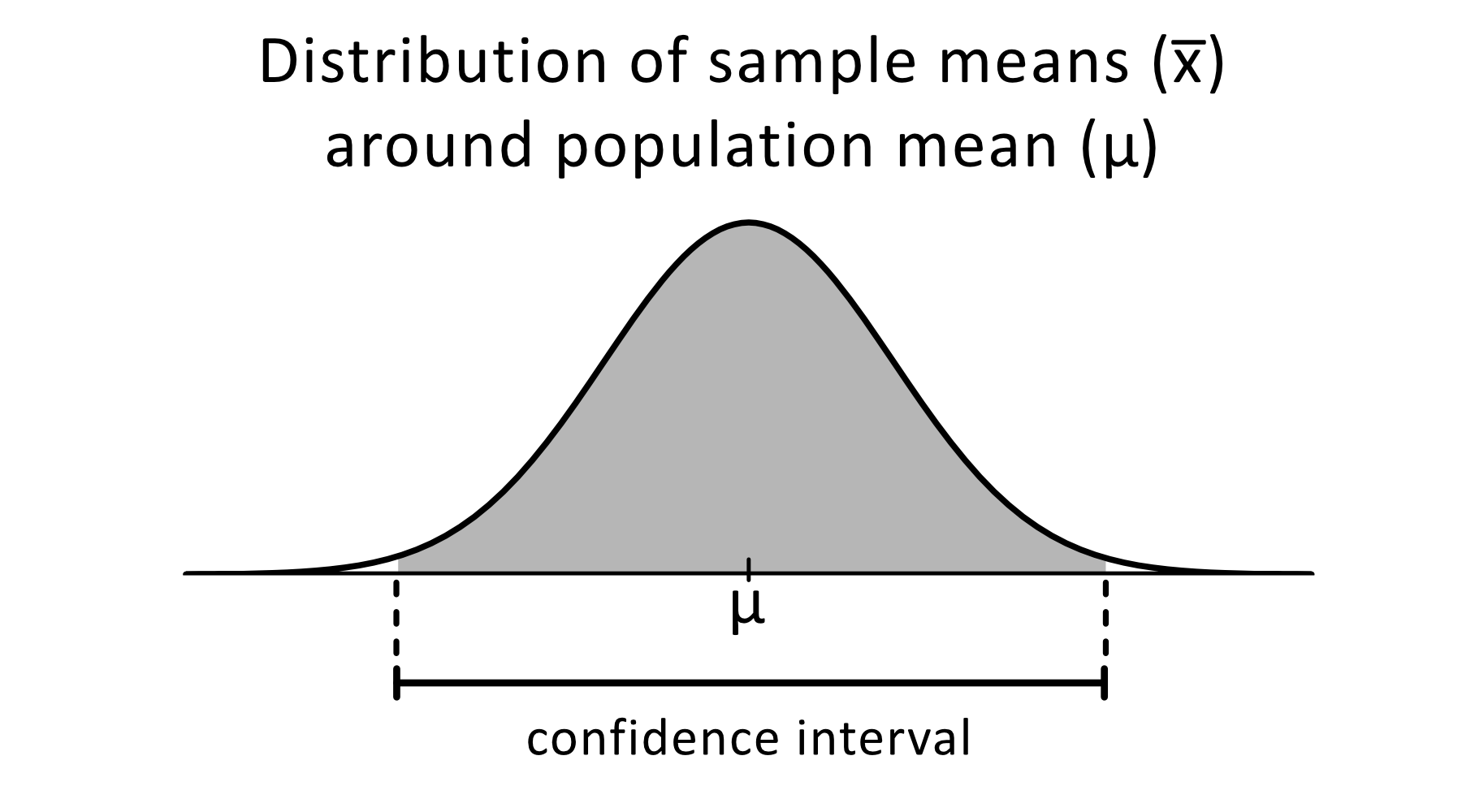# 99% Confidence Interval Calculator

Created by Aleksandra Zając, MD
Reviewed by Rijk de Wet
Last updated: Feb 02, 2023

If you've got a data set and want to perform statistical calculations on it, our 99% confidence interval calculator is a good place to start. We've got you covered — not only if you want to know how to find this 99% confidence interval, but also to learn:

• What is Z-score;
• What does confidence interval even mean; and
• How to find a margin of error for a 99% confidence interval.

## What is a confidence interval?

A confidence interval is the range of values you expect your parameter to fall in if you repeat a test multiple times. Let's see an example that puts confidence intervals into real life.

Becky sells homemade muffins, and she wants to check the average weight of her baked goods. She found that 99% of her muffins weigh between 121 and 139 grams (4.27–4.9 oz), while one muffin came out of the oven at a whopping 160 grams (5.64 oz) — much bigger than expected!

The 99% confidence interval of Becky's muffins' weights is the range of 121 to 139 g. And so, when selling muffins, she can be 99% sure that any muffin she baked weighs between 121 and 139 g. But 1% of the time, she might accidentally produce a chonky muffin (or a tiny one!)

## How do I find a 99% confidence interval?

Estimating confidence intervals is a bit of work, but we'll walk you through the process step by step.

1. First, you need to know:

• $n$ — the size of your sample (the number of measurements taken);
• $μ$ — the mean (average) of the measurements; and
• $σ$ — the standard deviation of the measurements.
2. Now, you can calculate standard error and margin of error with the formulas:

$\footnotesize \qquad \text{SE} = \frac{σ}{\sqrt{n}} \\[6pt] \qquad \text{ME} = \text{SE} × Z(0.99)$

where:

• $SE$ — the standard error;
• $ME$ — the margin of error;
• $Z(0.99)$ — the z-score corresponding to the chosen confidence level (which you'll find in statistical tables).
1. Add and subtract the margin of error value from the mean to obtain your confidence interval. It is the range between these lower and upper bounds.
$\footnotesize \qquad\text{upper bound} = \mu + ME \\ \qquad\text{lower bound} = \mu - ME$

## How to use 99% confidence interval calculator

Now that you know how complicated it is to count confidence intervals on your own, you know what's going on behind the scenes with our 99% confidence interval calculator! Here's how to use it:

1. Fill in the sample mean (x̅) in the first row.
2. Enter the standard deviation (s).
3. Enter the sample size (n).
4. Your confidence level is already filled in (99%), but keep in mind you can change it anytime.
5. The Z-score will update automatically as you decide on the confidence interval.
6. And that's it! At the bottom of the calculator, you'll see:
• A chart describing your data;
• The confidence interval range — with a lower and upper bound indicated; and
• The margin of error.

## Confidence interval tools

If you're interested in statistics, you might find those tools helpful:

## FAQ

### What is the Z-score for a 99% confidence interval?

The z-score for a two-sided 99% confidence interval is 2.807, which is the 99.5-th quantile of the standard normal distribution N(0,1).

### How to find the margin of error for a 99% confidence interval?

To find the margin of error for a 99% confidence interval:

1. Find Z(0.99) (the z-score for 99% confidence) in the statistical table.
Z(0.99) = 2.576
2. Calculate the standard error with the formula SE = σ/√n, where σ is the standard deviation and n is the sample size.
3. Multiply Z(0.99) by the standard error to obtain the margin of error, ME.
ME = Z(0.99) × SE
Aleksandra Zając, MDSample mean (x̅)
Standard deviation (s)
Sample size (n)
Confidence level
%
Z-score (Z)
σ99% of samples contain the population mean (μ) within the confidence interval x̅ ± ME.
Confidence interval
Lower bound
Upper bound
Margin of error (ME)
People also viewed…

### Histogram

The histogram calculator is a histogram maker and a lesson on histograms, all in one. Let's explore what is a histogram, some examples, and the differences between a histogram vs a bar chart.

### Millionaire

This millionaire calculator will help you determine how long it will take for you to reach a 7-figure saving or any financial goal you have. You can use this calculator even if you are just starting to save or even if you already have savings.

### Negative binomial distribution

The negative binomial distribution calculator calculates what is the probability according to the Pascal distribution.